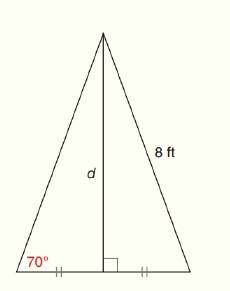Chapter 11.CR, Problem 2CRElementary Geometry For College St...

7th Edition
Alexander + 2 others
ISBN: 9781337614085

Solutions

Chapter
SectionElementary Geometry For College St...

7th Edition
Alexander + 2 others
ISBN: 9781337614085
Textbook Problem

In Review Exercises 1 to 4, state the ratio needed, and use it to find the measure of the indicated line segment to the nearest tenth of a unit.To determine

To find:

The measure of the of the indicated line segment to the nearest tenth of a unit.

Explanation

Procedure used:

In any right triangle ABC with mA=θ we have the following ratios.

sinθ=OppositeHypotenuse

Calculation:

Given:

The above figure is a triangle which has two right angled triangles. Let us name the given triangle as follows.

Thus,

In ABD

mABD=70°,

In  ACD

AC=8 ft.

In the given triangle ABC,

BD=CD=x (say)

From the given figure it is clear that the triangle ABD and triangle ACD are similar since,

Thus,

mABC=mACD=70°

mACD=70°

Thus, in ACD we have the acute angle mACD=70°

Length of the hypotenuse =8 ft

Still sussing out bartleby?

Check out a sample textbook solution.

See a sample solution

The Solution to Your Study Problems

Bartleby provides explanations to thousands of textbook problems written by our experts, many with advanced degrees!

Get Started

In Exercises 1-6, simplify the expression. (3x)+(x+3)3

Calculus: An Applied Approach (MindTap Course List)

let f(x) = x3 + 5, g(x) = x2 2, and h(x) = 2x + 4. Find the rule for each function. 6. fgh

Applied Calculus for the Managerial, Life, and Social Sciences: A Brief Approach

Fill in each blank: 5lb3oz=oz

Elementary Technical Mathematics

The length of the curve given by x = 3t2 + 2, y = 2t3, is:

Study Guide for Stewart's Single Variable Calculus: Early Transcendentals, 8th